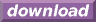#Calcute: freeware calculator

## Negative (neg, -)

A real number less than zero is called negative. A negative number is expressed by placing a negative (-) or "minus" sign before the number. This notation suggests that a positive number is being subtracted from an implicit zero: -4 = 0-4 for example. Calcute implements function neg to convert a positive number into its negative counterpart and, conversely, to convert a negative number into its positive counterpart. For a real number x, neg(x) = -x. This is equivalent to using the negative sign before the value being converted.

```neg(2)
-2
```